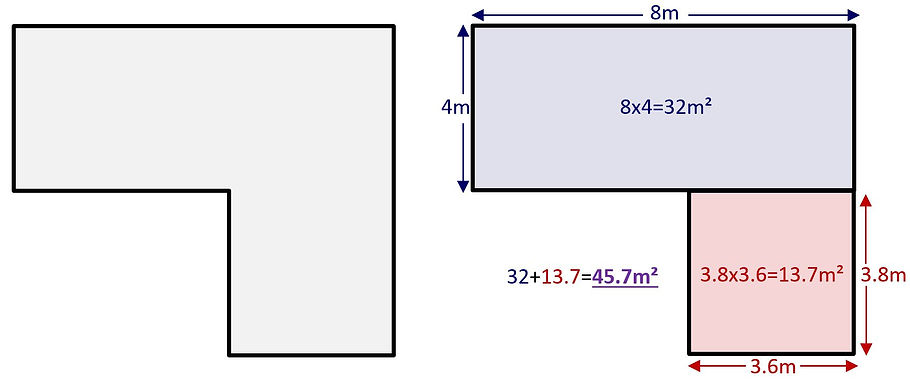top of page# ULTRA# Specification - Measuring

Before you can accurately calculate the costs of an installation you need to measure the application area. To measure a square or rectangular area you simply measure the length and the width of the area. These two measurements are then multiplied one by another.Example: If a rectangular driveway measures 8m long and 4m wide, the total area is 32m² (8x4=32).

If the application area has an irregular shape it is easier to draw a sketch of the layout and then identify areas which can be split into separate squares or rectangles. Each of these areas can then be measured in the way explained above and the totals simply added together.The next stage is to use the calculated total m² area to work out the material requirements. To do this simply use the table on the next page to find the name of your selected aggregate blend and the required application depth.

Example: A StarScape ULTRA surface laid at 20mm depth using the Mimosa aggregate blend = 3.3m²

This number (3.3m²) is the total m² each 100kg resin/aggregate mix will cover. Next simply take your total area and divide it by this number:

45.7÷3.3=13.8

Round up the number (13.8=14) and this is the number of kits you will require. As each kit is mixed with 100kg of aggregate, simply multiply the number by 100 for the weight of aggregate required (14x100=1400).Example: Calculating the material requirements for an area of 175m² using the StarScape ALCOR aggregate blend at a minimum depth of 25mm:

Area ÷ Coverage Rate = Resin Kits        175 ÷ 2.6 = 67.3

Resin Kits rounded up                                 67.3 = 68

Resin Kits x Mix size = Aggregate          68 x 100 = 6800

The total material requirements for the ALCOR aggregate blend laid at a minimum depth of 25mm would be 68 kits of StarScape ULTRA and 6800kg of aggregate.

bottom of page